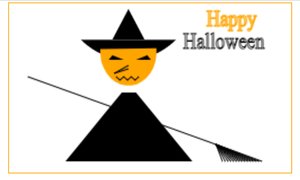Open In App

# Design a Happy Halloween Porter using HTML and CSS

In this article, we will make a ‘Happy Halloween Poster’  using HTML canvas, along with understanding some of the basic functions provided by canvas in Javascript. Canvas is a digital technique to draw graphics via JavaScript & the elements of the canvas can be used as a container for graphics. Using this, we can draw a line, arc, rectangle, or fill color in any closed figure or write text as well. By default, a canvas has no border and no content. The below are functions defined that can be used to draw the canvas:

• lineTo(x, y): This function is used to draw a line from the current location of the canvas pointer to a specified point that has x and y coordinates.
• arc(x, y, a1, a2, direction): We can utilize this function to draw arcs which can be half circle, full circle, or any part of the circle depending on starting angle and ending angle given which is a1 and a2 in radians. Also, we can specify coordinates of the center of the circle which is x and y.
• moveTo(x, y): There might be times when we have to move the pen from the current point to some other point but without drawing any line or anything, so this function can be used to just move the pen to a point whose coordinates will be x and y.
• strokeText(“text”, x, y): This function can be used to insert text inside canvas at the specified point with coordinates x and y.
• stroke(), fill(): All above functions are of no use if we do not use these functions to command the canvas when to draw/stroke something and when to fill colors in a figure.
• beginPath(), closePath(): These functions need to be used to tell the canvas when to start/end a path.

Properties of canvas:

• strokeStyle: It defines the color of the line.
• fillStyle: It defines the color used to fill figures.
• lineJoin: It defines the way lines will be at the joints.
• lineCap: It defines how the ends of a line should look like.
• font: It defines the font style and size of the text.

We will utilize the above functions & properties the design Halloween.

Example: This example describes the use of the canvas in HTM & Javascript.

## HTML

 ```<``html` `lang``=``"en"``>`` ` `<``head``>``    ``<``meta` `charset``=``"UTF-8"` `/>``    ``<``meta` `http-equiv``=``"X-UA-Compatible"` `          ``content``=``"IE=edge"` `/>``    ``<``meta` `name``=``"viewport"` `          ``content="``width``=``device``-width,``                   ``initial-scale``=``1``.0" />``    ``<``style``>``    ``canvas {``        ``border: 3px solid orange;``        ``height: 600px;``        ``width: 1000px;``        ``margin-left: 10%;``    ``}``    ````    ``<``title``>Halloween```` ` `<``body``>``    ``<``canvas``> ``    ``<``script``>``        ``const ctx = ``            ``document.querySelector("canvas").getContext("2d");``        ``ctx.beginPath();``        ``ctx.lineCap = "round";``        ``ctx.lineJoin = "round";``        ``ctx.moveTo(20, 65);``        ``ctx.lineTo(220, 125);``        ``for(let i = 227; i <= 269; i += 3) {``            ``ctx.moveTo(220, 125);``            ``ctx.lineTo(i, 140);``        ``}``        ``ctx.stroke();``        ``ctx.closePath();``        ``ctx.beginPath();``        ``ctx.fillStyle = "black";``        ``ctx.moveTo(60, 140);``        ``ctx.lineTo(120, 79);``        ``ctx.lineTo(135, 79);``        ``ctx.lineTo(195, 140);``        ``ctx.lineTo(60, 140);``        ``ctx.fill();``        ``ctx.closePath();``        ``ctx.beginPath();``        ``ctx.arc(127, -30, 80, Math.PI / 3.5, (Math.PI * 3) / 4.2);``        ``ctx.fill();``        ``ctx.moveTo(127, 5);``        ``ctx.lineTo(105, 40);``        ``ctx.lineTo(149, 40);``        ``ctx.lineTo(127, 5);``        ``ctx.fill();``        ``ctx.closePath();``        ``ctx.beginPath();``        ``ctx.fillStyle = "orange";``        ``ctx.arc(127, 43, 30, -Math.PI / 30, (-Math.PI * 35) / 36, false);``        ``ctx.fill();``        ``ctx.closePath();``        ``ctx.beginPath();``        ``ctx.lineCap = "round";``        ``ctx.lineJoin = "round";``        ``ctx.moveTo(127, 55);``        ``ctx.lineJoin = "round";``        ``ctx.lineCap = "round";``        ``ctx.lineTo(112, 62);``        ``ctx.lineTo(127, 58);``        ``ctx.stroke();``        ``ctx.closePath();``        ``ctx.beginPath();``        ``ctx.fillStyle = "black";``        ``ctx.moveTo(122, 50);``        ``ctx.lineTo(110, 46);``        ``ctx.lineTo(106, 50);``        ``ctx.lineTo(122, 50);``        ``ctx.fill();``        ``ctx.closePath();``        ``ctx.beginPath();``        ``ctx.fillStyle = "black";``        ``ctx.moveTo(135, 50);``        ``ctx.lineTo(147, 46);``        ``ctx.lineTo(151, 50);``        ``ctx.lineTo(135, 50);``        ``ctx.fill();``        ``ctx.closePath();``        ``ctx.beginPath();``        ``ctx.lineCap = "round";``        ``ctx.lineJoin = "round";``        ``ctx.moveTo(114, 65);``        ``ctx.lineTo(118, 68);``        ``ctx.lineTo(122, 65);``        ``ctx.lineTo(126, 68);``        ``ctx.lineTo(130, 65);``        ``ctx.lineTo(134, 68);``        ``ctx.lineTo(138, 65);``        ``ctx.stroke();``        ``ctx.closePath();``        ``ctx.beginPath();``        ``ctx.strokeStyle = "orange";``        ``ctx.font = "20px Serif";``        ``ctx.strokeText("Happy", 210, 20);``        ``ctx.strokeStyle = "black";``        ``ctx.strokeText("Halloween", 185, 40);``    `````` ` ``

Output: